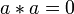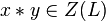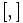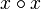# Linear halving generalization of Baer correspondence

## Statement

This is a generalization of the Baer correspondence between some Lie rings of class at most two and some groups of class at most two. Specifically, it is a correspondence:

Lie rings of class at most two where the Lie bracket arises by doubling the Lie bracket of a Lie ring of class at most two$\leftrightarrow$ Groups of class at most two where the commutator arises by squaring the commutator of a group of class at most two

Note that this correspondence is a little different from the Baer correspondence and the LCS-Baer correspondence (which are both special cases of it) in the following important sense: the specific element-by-element correspondence depends on the choice of the halved linear map. Different choices of the halved linear map can give different bijections, even qualitatively different ones. However, it turns out that the isomorphism class of the Lie ring completely determines that of the group and vice versa.

### From Lie ring to group

Suppose$L$ is a Lie ring of class at most two. Suppose we find a function:$*:L \times L \to L$

satisfying the following conditions:

•$*$ is biadditive, i.e., it is$\mathbb{Z}$-bilinear. In other words,$a * (b + c) = (a * b) + (a * c)$ and$(a + b) * c = (a * c) + (b * c)$ for all$a,b,c \in L$.
•$*$ is alternating, i.e.,$a * a = 0$ for all$a \in L$. This also implies that$*$ is skew symmetric, i.e.,$a * b = -(b * a)$ for all$a,b \in L$.
•$2(x * y) = [x,y]$ for all$x,y \in L$.
•$x * y \in Z(L)$ for all$x,y \in L$.
•$x * y = 0$ whenever$x \in L$ and$y \in Z(L)$.

Equivalently, we can state the above as:$*$ is a Lie bracket on$L$ that gives$L$ the structure of a Lie ring of class at most two, and such that$2(*) = [ , ]$.

Then, we can define the Lie group corresponding to$L$ by the multiplication$\cdot$:

Operation How it's denoted Definition in terms of Lie ring operations
Group multiplication$x \cdot y$$x \cdot y := x + y + (x * y)$
Identity element$e$$e := 0$
Inverse$x^{-1}$$x^{-1} := -x$
Group commutator$[x,y]$$[x,y] := [x,y]$ where the right side is the Lie bracket

### From group to Lie ring

Suppose$G$ is a group of class at most two. Suppose we find a function:$\circ:G \times G \to G$

satisfying the following conditions:

•$(x \circ y)^2 = [x,y]$ for all$x,y \in G$, where$[,]$ denotes the commutator in the group.
•$x \circ x$ is the identity element for any$x \in G$.
•$x \circ y = (y \circ x)^{-1}$ for all$x,y \in G$.
•$\circ$ is a bihomomorphism, i.e., it is a homomorphism in each coordinate.
•$x \circ y \in Z(G)$ for all$x,y \in G$.
•$x \circ y$ is the identity element if$x \in G$ and$y \in Z(G)$.

Then we can define the Lie ring corresponding to$G$ by the operation. Note that$x \circ y$ is central and hence multiplying or dividing by it on the left or the right yields the same results:

Operation How it's denoted Definition in terms of Lie ring operations
Lie ring addition$x + y$$x + y := xy(x \circ y)^{-1}$
Zero element$0$ same as multiplicative identity of the group
Additive inverse$-x$$-x := x^{-1}$
Lie bracket$[x,y]$$[x,y] := xyx^{-1}y^{-1}$, i.e., the right side is the commutator. Note that because we're in class two, the left/right convention issue does not matter.

## Particular cases

The Baer correspondence and the LCS-Baer correspondence both arise as special cases of this setup. In both cases, there is a natural choice of the halving map:

• For the Baer correspondence, there is a unique half for every element in the Lie ring or group.
• For the LCS-Baer correspondence, there is a unique half for every element in the derived subgroup/subring, where uniqueness of half is only within the derived subgroup/subring.

The linear halving generalization is somewhat more general in the following senses:

• First, even in cases where the Baer or LCS-Baer correspondence applies, the linear halving generalization can give other correspondences. This arises when the group has 2-torsion. For instance, for a nontrivial abelian 2-group, we can construct many nonzero halvings of the commutator map, which is the zero map. However, the uniqueness theorem for generalizations of Baer correspondence basically says that regardless of the choice of linear halving, the groups that we get as a result are all isomorphic to each other.
• Second, there are situations where the Baer correspondence and LCS-Baer correspondence do not work but a linear halving generalization does work. The smallest example of this sort is SmallGroup(64,57).